# 纯干货：深度学习实现之空间变换网络-part1

+关注继续查看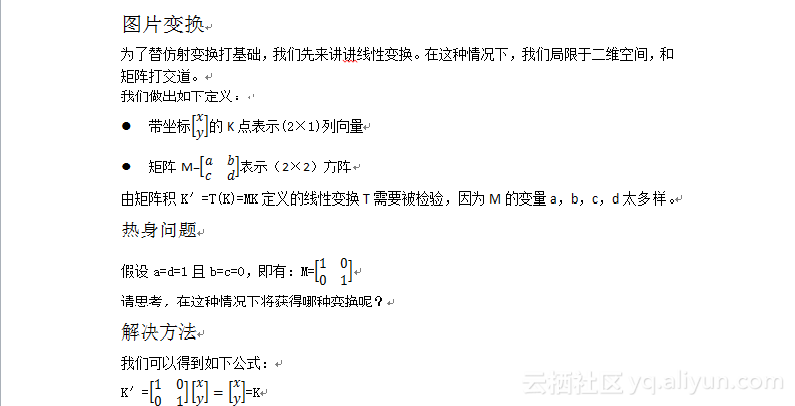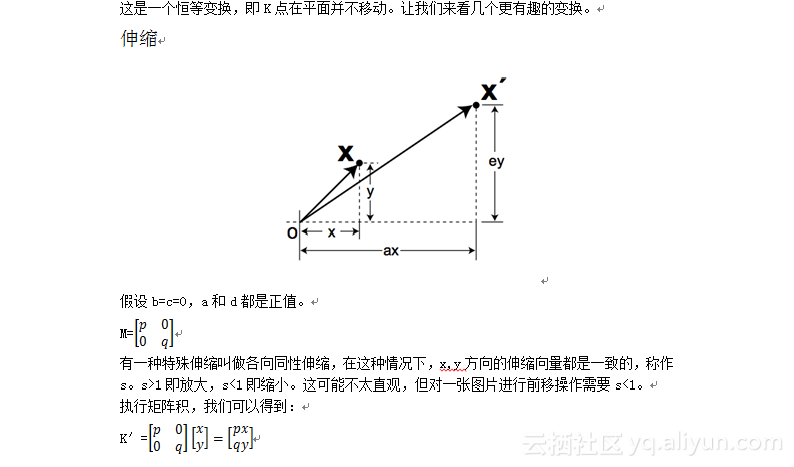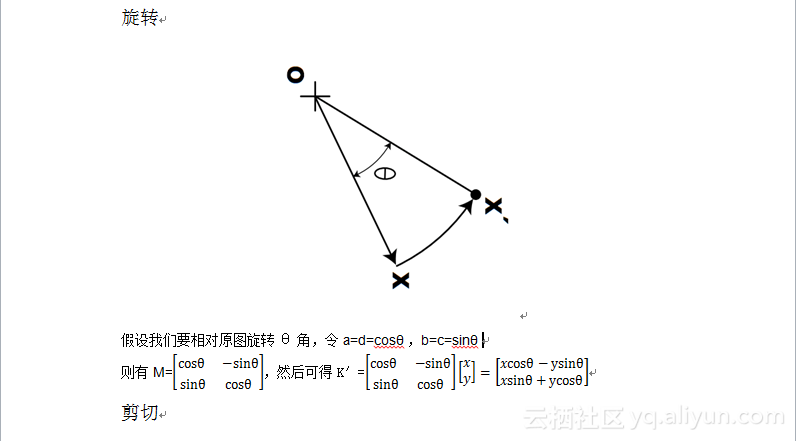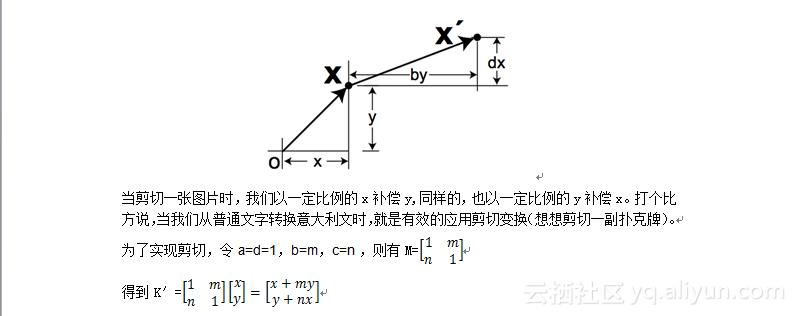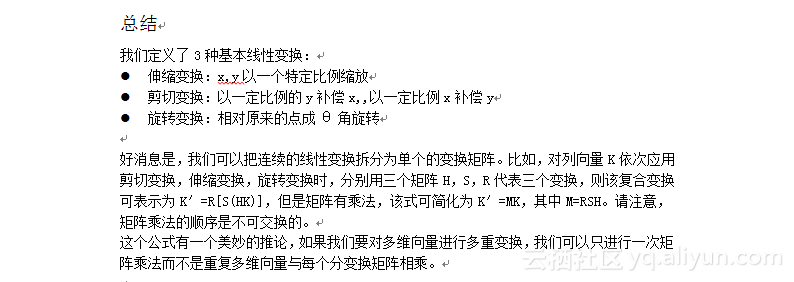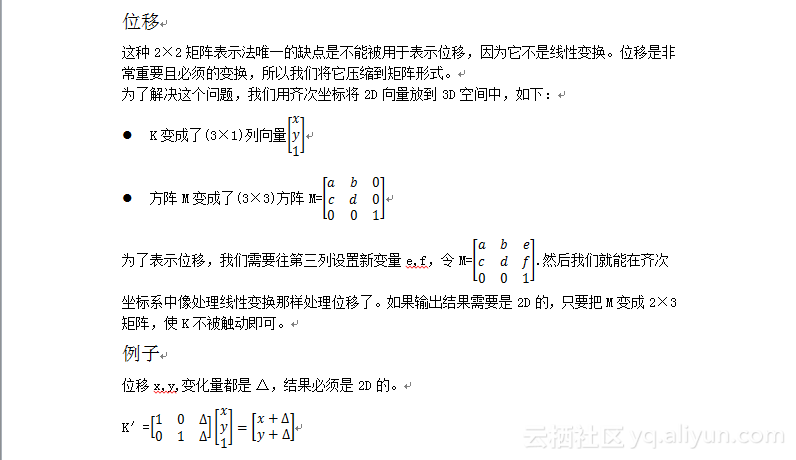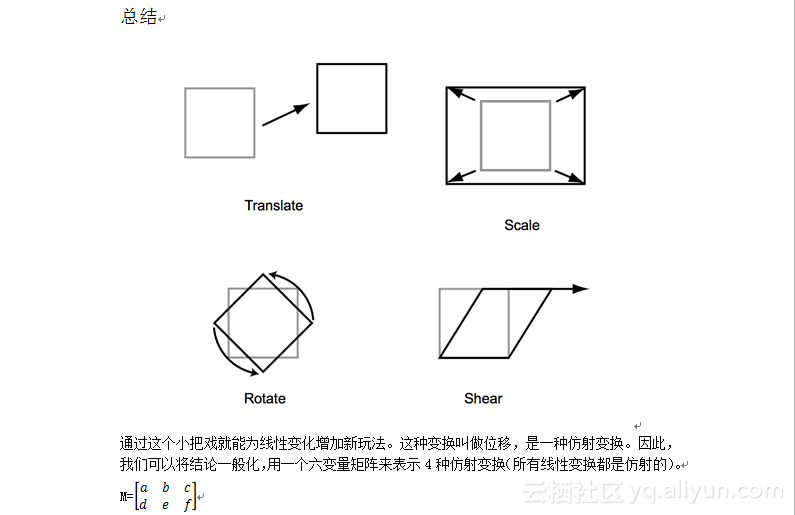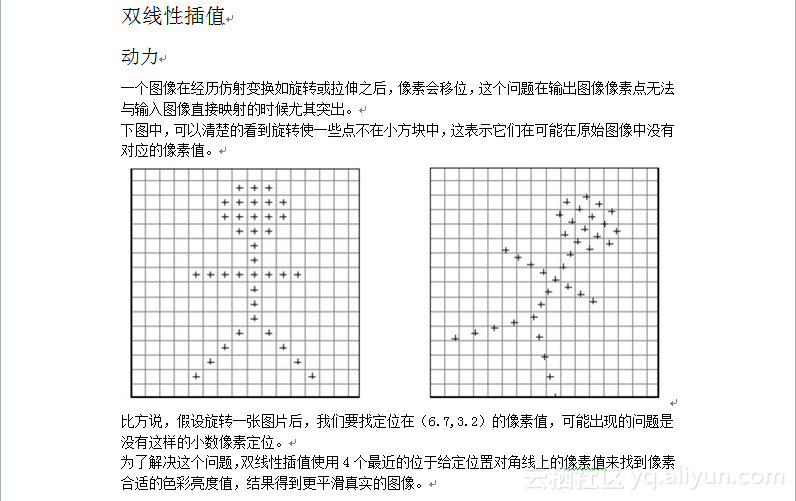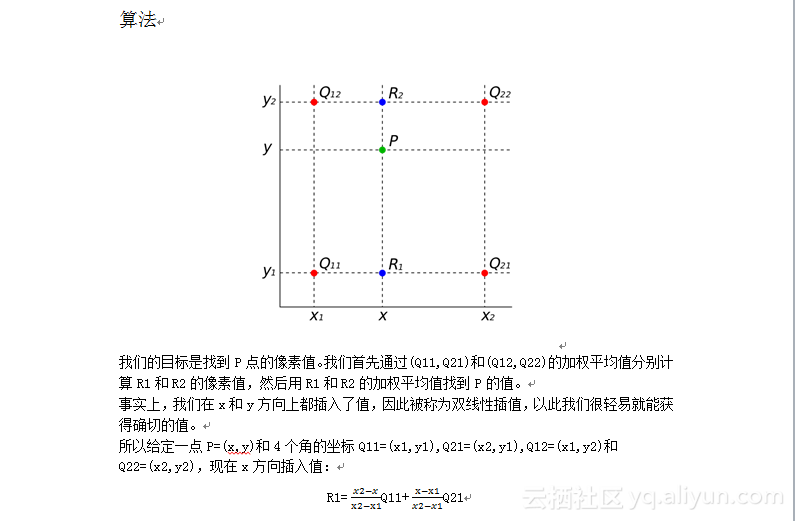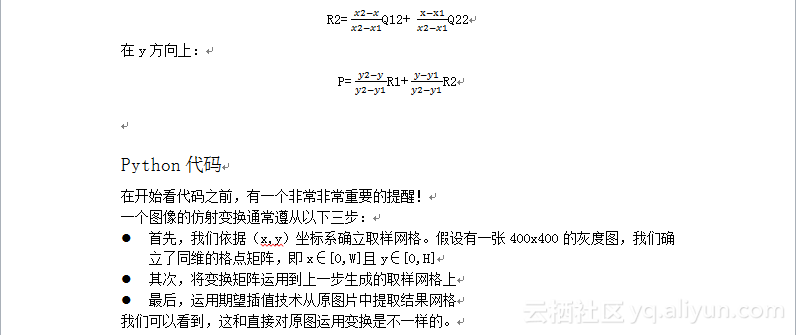import numpy as np

from PIL import Image

# params

DIMS = (400, 400)

CAT1 = 'cat1.jpg'

CAT2 = 'cat2.jpg'

# load both cat images

img1 = load_img(CAT1, DIMS)

img2 = load_img(CAT2, DIMS, view=True)

# concat into tensor of shape (2, 400, 400, 3)

input_img = np.concatenate([img1, img2], axis=0)

# dimension sanity check

print("Input Img Shape: {}".format(input_img.shape))

# grab shape

num_batch, H, W, C = input_img.shape

# initialize M to identity transform

M = np.array([[1., 0., 0.], [0., 1., 0.]])

# repeat num_batch times

M = np.resize(M, (num_batch, 2, 3))

（再次声明：如果要包含位移，仿射变换的通用矩阵是2×3的）

# create normalized 2D grid

x = np.linspace(-1, 1, W)

y = np.linspace(-1, 1, H)

x_t, y_t = np.meshgrid(x, y)

# reshape to (xt, yt, 1)

ones = np.ones(np.prod(x_t.shape))

sampling_grid = np.vstack([x_t.flatten(), y_t.flatten(), ones])

# repeat grid num_batch times

sampling_grid = np.resize(sampling_grid, (num_batch, 3, H*W))

# transform the sampling grid i.e. batch multiply

batch_grids = np.matmul(M, sampling_grid)

# batch grid has shape (num_batch, 2, H*W)

# reshape to (num_batch, height, width, 2)

batch_grids = batch_grids.reshape(num_batch, 2, H, W)

batch_grids = np.moveaxis(batch_grids, 1, -1)

x_s = batch_grids[:, :, :, 0:1].squeeze()

y_s = batch_grids[:, :, :, 1:2].squeeze()

# rescale x and y to [0, W/H]

x = ((x_s + 1.) * W) * 0.5

y = ((y_s + 1.) * H) * 0.5

# grab 4 nearest corner points for each (x_i, y_i)

x0 = np.floor(x).astype(np.int64)

x1 = x0 + 1

y0 = np.floor(y).astype(np.int64)

y1 = y0 + 1

(注意：我们只能用ceiling函数而不是增量1)

# make sure it's inside img range [0, H] or [0, W]

x0 = np.clip(x0, 0, W-1)

x1 = np.clip(x1, 0, W-1)

y0 = np.clip(y0, 0, H-1)

y1 = np.clip(y1, 0, H-1)

# look up pixel values at corner coords

Ia = input_img[np.arange(num_batch)[:,None,None], y0, x0]

Ib = input_img[np.arange(num_batch)[:,None,None], y1, x0]

Ic = input_img[np.arange(num_batch)[:,None,None], y0, x1]

Id = input_img[np.arange(num_batch)[:,None,None], y1, x1]

# calculate deltas

wa = (x1-x) * (y1-y)

wb = (x1-x) * (y-y0)

wc = (x-x0) * (y1-y)

wd = (x-x0) * (y-y0)

wa = np.expand_dims(wa, axis=3)

wb = np.expand_dims(wb, axis=3)

wc = np.expand_dims(wc, axis=3)

wd = np.expand_dims(wd, axis=3)

# compute output

out = wa*Ia + wb*Ib + wc*Ic + wd*Id

plt.imshow(out)

plt.show()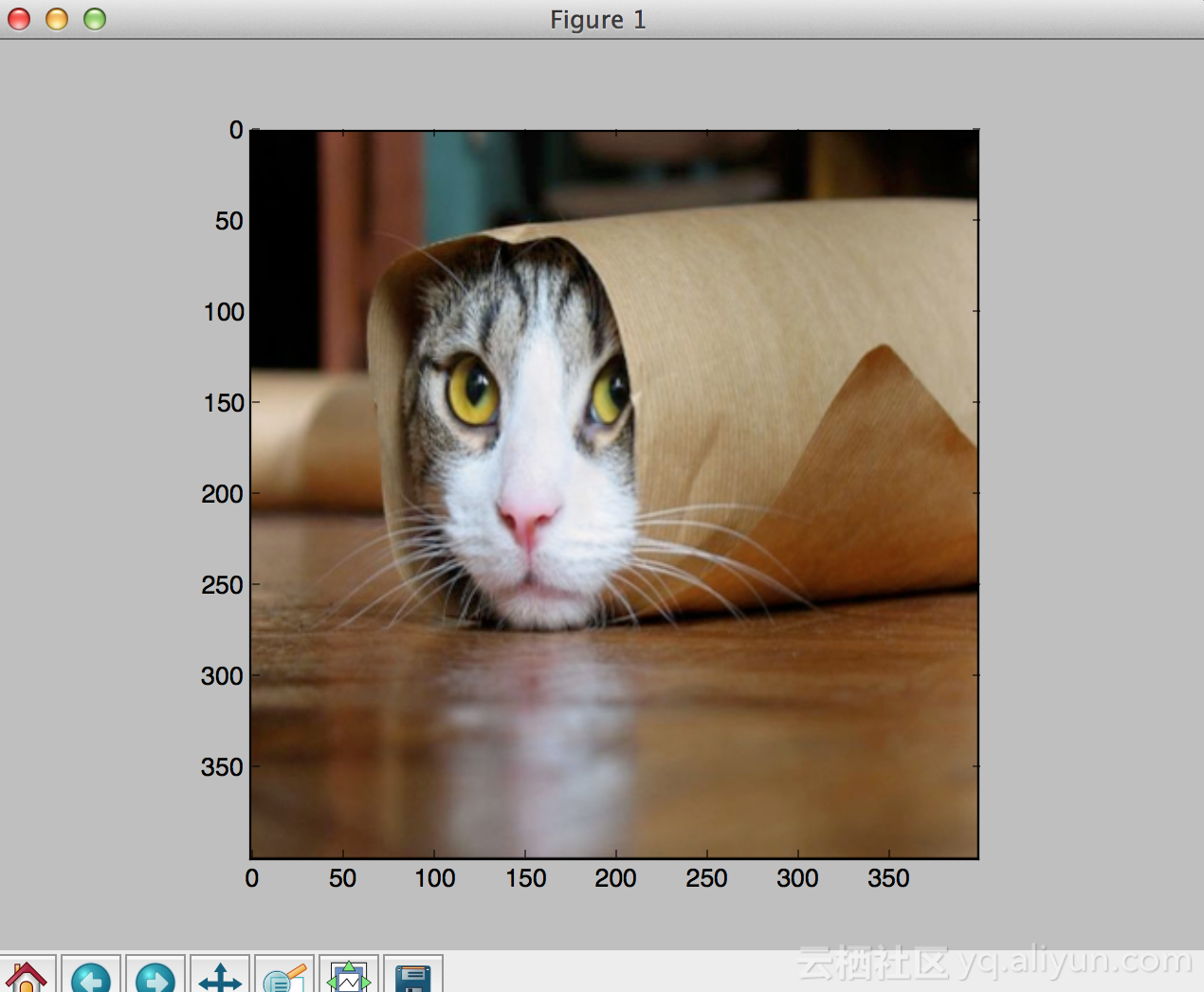M = np.array([[1., 0., 0.5], [0., 1., 0.]])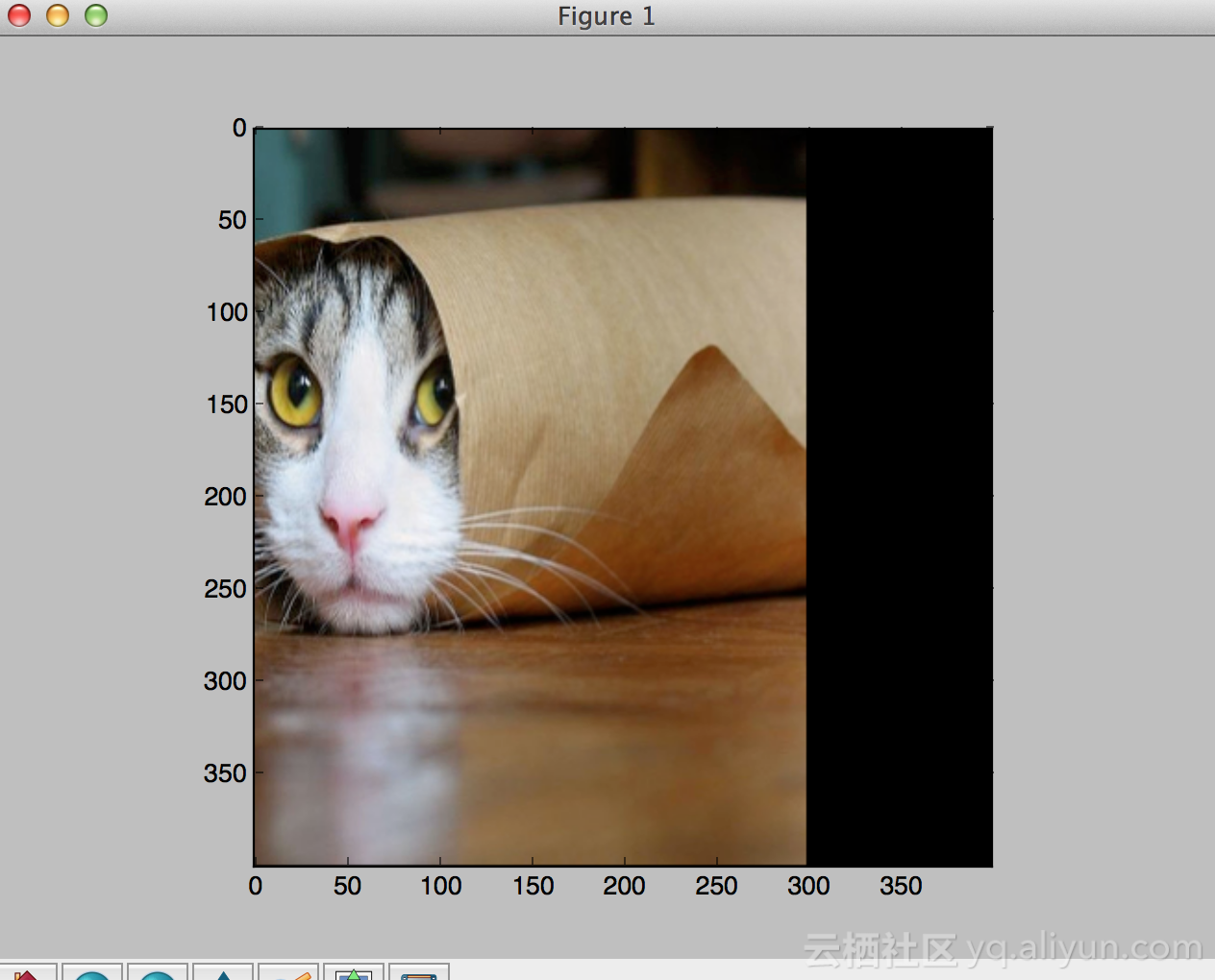M = np.array([[0.707, -0.707, 0.], [0.707, 0.707, 0.]])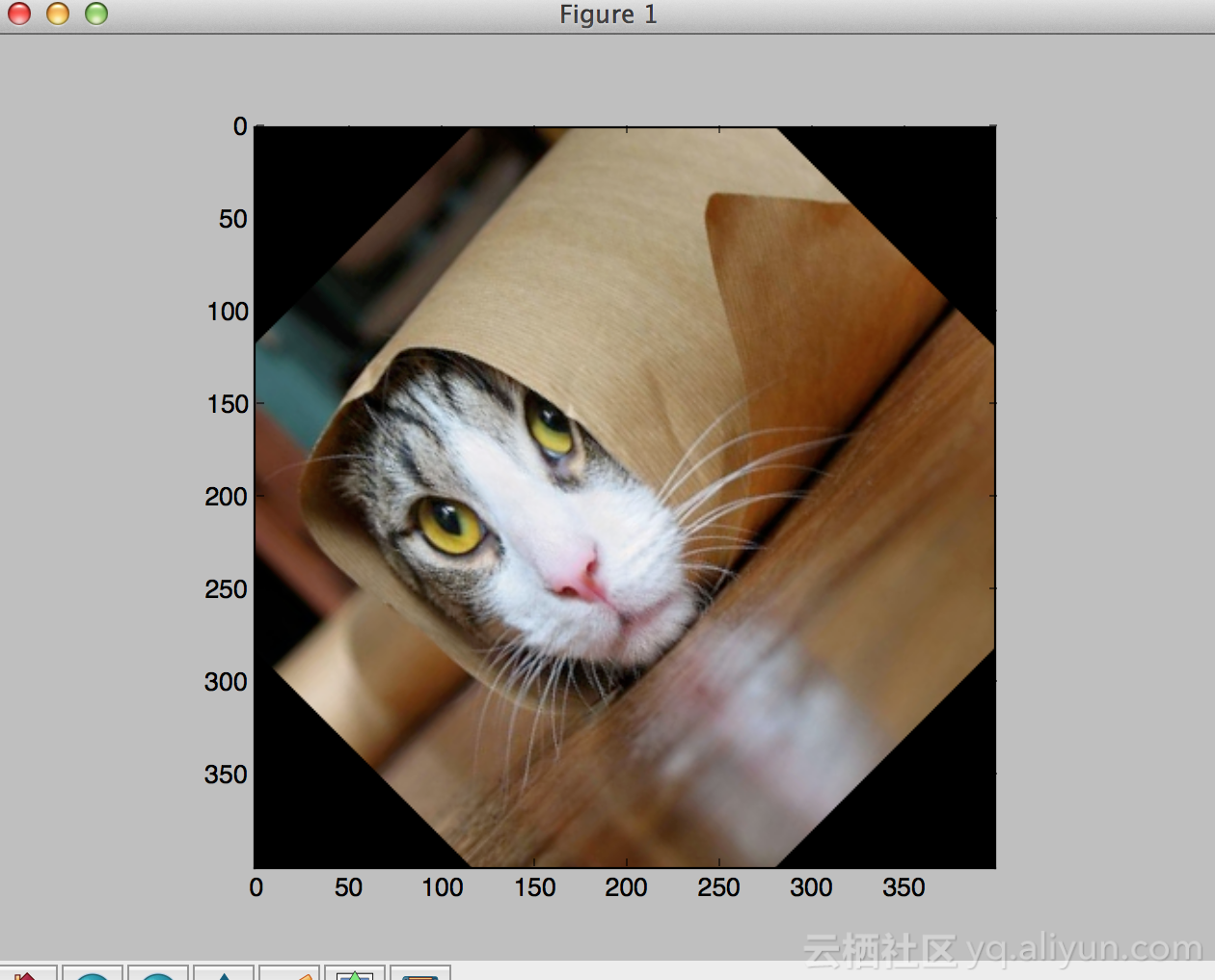Â· Bilinear Interpolation Wikipedia

Â· Bilinear Interpolation

Â· Matrix Transformations PDF

Â· Bilinear Interpolation Code

# 文章原标题《Deep Learning Paper Implementations: Spatial Transformer Networks - Part I》，作者：Kevin Zakka，译者：NancyWang#yyds干货盘点 前端歌谣的刷题之路-第十一题-伪类选择器
#yyds干货盘点 前端歌谣的刷题之路-第十一题-伪类选择器
10 087 03286 01699 0jenkins~集群分发功能的具体实现
1492 01489 0902 01182 013705 0
【方向】

706

5